## Figures index

#### Rafik Salloum, Mohammad Reza Arvan, Bijan Moaveni

Automatic Control and Information Sciences. 2014, 2(3), 53-58 doi:10.12691/acis-2-3-2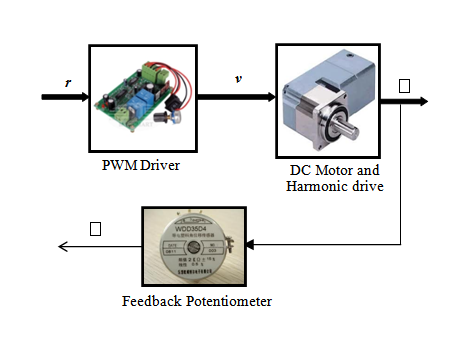• Figure 1. EMA harmonic drive scheme• Figure 2. Measured data and identified model for the EMA harmonic drive system• Figure 3. EMA system in H framework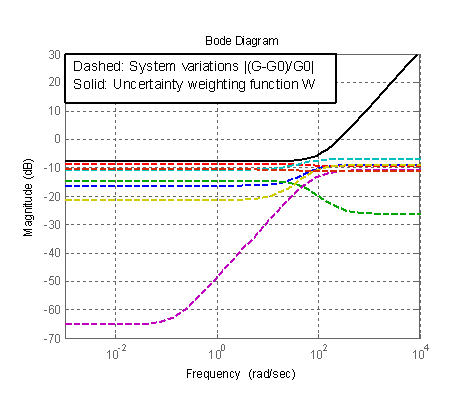• Figure 4. Uncertainty and weighting function frequency responses; simulation• Figure 5. Closed loop sensitivity, complementary sensitivity, robustness and performance functions with Hcontroller; simulation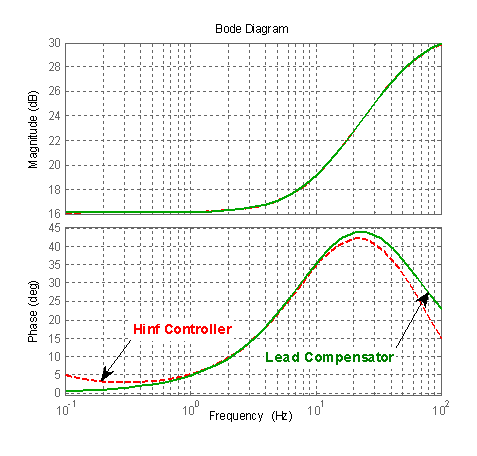• Figure 6. H controller and the reduced one; simulation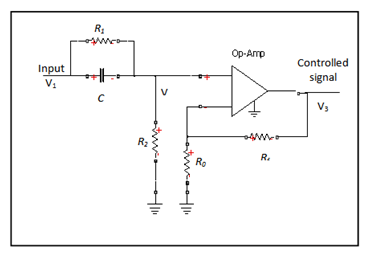• Figure 7. Designed compensator circuit• Figure 8. Step responses of nominal and uncertain closed loop EMA with the derived lead compensator; simulation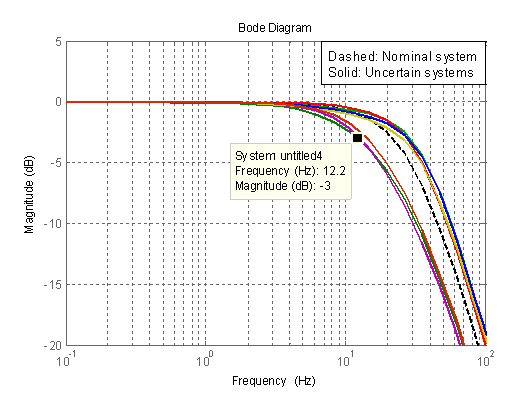• Figure 9. Closed loop frequency response for nominal and uncertain EMA with the lead compensator; simulation• Figure 10. Control signals with H controller and the derived lead compensator; simulation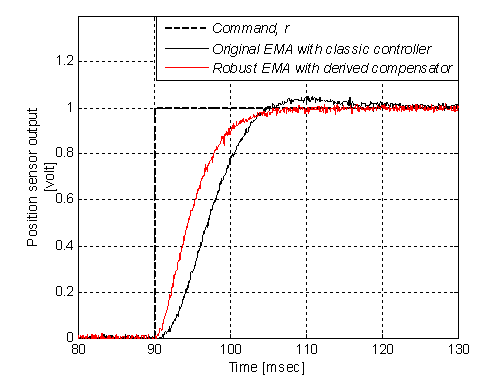• Figure 11. Measured step for original and robust EMA systems; experiment